# Class 10 Maths Chapter 10 NCERT Solutions Circle

In this page we have Class 10 Maths Chapter 10 NCERT Solutions Circle for Exercise 10.2 . Hope you like them and do not forget to like , social share and comment at the end of the page.
Important things to remember
1) The tangent at any point of a circle is perpendicular to the radius through the point of contact
2) Length of Length
The length of the segment of the tangent from the external point P and the point of contact with the circle is called the length of the tangent from point to the circle
3)  The length of the two tangents drawn from the external point to the circle are equal
In Q.1 to 3, choose the correct option and give justification.
Question 1.
From a point Q, the length of the tangent to a circle is 24 cm and the distance of Q from the centre is 25 cm. The radius of the circle is
(A) 7 cm
(B) 12 cm
(C) 15 cm
(D) 24.5 cm
Solution
We know that The line drawn from the centre of the circle to the tangent is perpendicular to the tangent.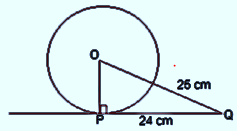∴ ΔOPQ is right angled triangle
OQ = 25 cm and PQ = 24 cm (Given)
By Pythagoras theorem in ΔOPQ,
(Hypotenuse)2 = (Perpendicular)2 + (Base)2
OQ2 = OP2 + PQ2
(25)= OP2 + (24)2
OP2 = 49
OP = 7 cm
Hence option (A) 7 cm.
Question 2.
In below figure, if TP and TQ are the two tangents to a circle with centre O so that ∠POQ = 110°, then ∠PTQ is equal to
(A) 60°
(B) 70°
(C) 80°
(D) 90°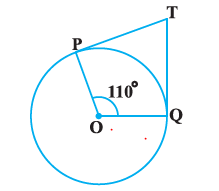Solution
OP and OQ are radii of the circle to the tangents TP and TQ respectively.
Therefore OP ⊥ TP and OQ ⊥ TQ
So ∠OPT = ∠OQT = 90°
Sum of all interior angles = 360°
∠PTQ + ∠OPT + ∠POQ + ∠OQT = 360°
∠PTQ + 90° + 110° + 90° = 360°
∠PTQ = 70°
Hence option (B) 70°.
Question 3.
If tangents PA and PB from a point P to a circle with centre O are inclined to each other at angle of 80°, then ∠POA is equal to
(A) 50°
(B) 60°
(C) 70°
(D) 80°
Solution
OA and OB are radii of the circle to the tangents PA and PB respectively.
There OA ⊥ PA and OB ⊥ PB
So ∠OBP = ∠OAP = 90°
Sum of all interior angles = 360°
∠AOB + ∠OBP + ∠OAP + ∠APB = 360°
∠AOB + 90° + 90° + 80° = 360°
∠AOB = 100°
Now,
In ΔOPB and ΔOPA,
AP = BP [Tangents from a point are equal]
OA = OB [Radii of the same circle]
OP = OP (Common side)
By SSS congruence condition
ΔOPB ≅ ΔOPA
Thus ∠POB = ∠POA
So, line OP bisect the angle ∠AOB
So ∠POA = ∠AOB /2 = 100/2 = 500
Hence option (A) 50°
Question 4
Prove that the tangents drawn at the ends of a diameter of a circle are parallel.
Solution
Let AB be a diameter of the circle. Two tangents PQ and RS are drawn at points A and B respectively.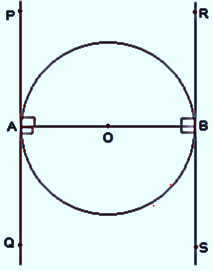We know that Radii of the circle to the tangents will be perpendicular to it.
Therefore OB ⊥ RS and OA ⊥ PQ
So ∠OBR = ∠OBS = ∠OAP = ∠OAQ = 90º
From the figure,
∠OBR = ∠OAQ (Alternate interior angles)
∠OBS = ∠OAP (Alternate interior angles)
Since alternate interior angles are equal, lines PQ and RS will be parallel.
Hence Proved that the tangents drawn at the ends of a diameter of a circle are parallel.
Question 5
Prove that the perpendicular at the point of contact to the tangent to a circle passes through the centre.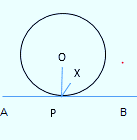Let us consider a circle with centre O. Let P be the point of contact of the tangent AB to the circle
We have to  prove that the line perpendicular to AB at P passes through centre O.
We will prove this by contradiction method.
Let us assume that the perpendicular to tangent at P does not pass through centre O. Let it pass through another point X. Join XP and OP.
Now as XP is perpendicular to the tangent
So ∠XPB =900
Now O is the center of the circle and P is the point of contact. We know that line joining the center and point of contact is the perpendicular to tangent
So ∠OPB =900
Therefore ∠XPB and ∠OPB are equal
∠XPB =∠OPB
But From the figure
∠OPB > ∠XPB
So, our assumption is not possible. This can be possible only when OP and XP line coincides
So, line perpendicular to AB at P passes through centre O.

Question 6
The length of a tangent from a point A at distance 5 cm from the centre of the circle is 4 cm. Find the radius of the circle.
Solution
AB is a tangent drawn on this circle from point A.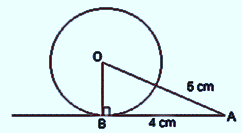∴ OB ⊥ AB
OA = 5cm and AB = 4 cm (Given)
By Pythagoras theorem in ΔABO,
(Hypotenuse)2 = (Perpendicular)2 + (Base)2
OA2 = AB+ BO2
5= 4+ BO2
BO2 = 9
BO = 3
So, The radius of the circle is 3 cm.
Question 7.
Two concentric circles are of radii 5 cm and 3 cm. Find the length of the chord of the larger circle which touches the smaller circle.
Solution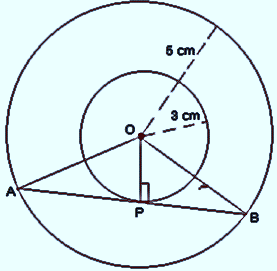Let the two concentric circles with centre O.
AB be the chord of the larger circle which touches the smaller circle at point P.
As AB is tangent to the smaller circle to the point P.
OP ⊥ AB
By Pythagoras theorem in ΔOPB,
(Hypotenuse)2 = (Perpendicular)2 + (Base)2
OB2 =  PB2 + OP2
52 = PB2 + 32
PB = 4
In ΔOAB,
Since OP ⊥ AB,
AP = PB (Perpendicular from the center of the circle  bisects the chord)
AB = 2PB = 2 × 4 = 8 cm

Question 8
A quadrilateral ABCD is drawn to circumscribe a circle as shown in below Figure. Prove that AB + CD = AD + BC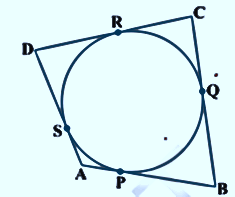Solution

From the figure, we observe that,
DR = DS (Tangents on the circle from point D) … (i)
AP = AS (Tangents on the circle from point A) … (ii)
BP = BQ (Tangents on the circle from point B) … (iii)
CR = CQ (Tangents on the circle from point C) … (iv)
DR + AP + BP + CR = DS + AS + BQ + CQ
(BP + AP) + (DR + CR) = (DS + AS) + (CQ + BQ)
CD + AB = AD + BC

Question 9
In below Figure, XY and X′Y′ are two parallel tangents to a circle with centre O and another tangent AB with point of contact C intersecting XY at A and X′Y′ at B. Prove that ∠ AOB = 90°.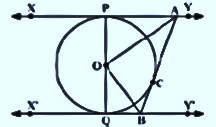Solution
We joined O and C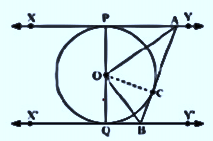In ΔOPA and ΔOCA,
OP = OC (Radii of the same circle)
AP = AC (Tangents from point A)
AO = AO (Common side)
∴ ΔOPA ≅ ΔOCA (SSS congruence criterion)
∠POA = ∠COA … (i)
Similarly,
ΔOQB ≅ ΔOCB
∠QOB = ∠COB … (ii)
Since POQ is a diameter of the circle, it is a straight line.
∴ ∠POA + ∠COA + ∠COB + ∠QOB = 180º
From equations (i) and (ii),
2∠COA + 2∠COB = 180º
∠COA + ∠COB = 90º
∠AOB = 90°

Question 10
Prove that the angle between the two tangents drawn from an external point to a circle is supplementary to the angle subtended by the line-segment joining the points of contact at the centre.
Solution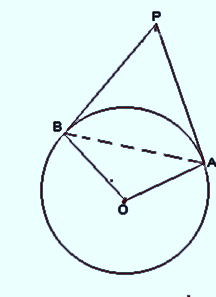Consider a circle with centre O. Let P be an external point from which two tangents PA and PB are drawn to the circle which are touching the circle at point A and B respectively and AB is the line segment, joining point of contacts A and B together such that it subtends ∠AOB at center O of the circle.
We know that Radii of the circle to the tangents will be perpendicular to it.
Therefore
OA ⊥ PA  and OB ⊥ PB
So  ∠OAP = 90°
and ∠OBP = 90°
Sum of all interior angles = 360º
∠OAP +∠APB +∠PBO +∠BOA = 360º
90º + ∠APB + 90º + ∠BOA = 360º
∠APB + ∠BOA = 180º
So, it is supplementary
Question 11
Prove that the parallelogram circumscribing a circle is a rhombus.
Solution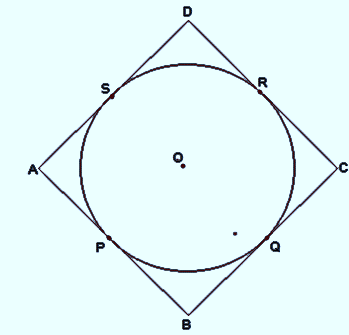Let ABCD is a parallelogram circumscribing a circle is a rhombus
AB = CD

Let the parallelogram touches the circle at P,Q,R and S
From the figure, we observe that,
DR = DS (Tangents to the circle at D)
CR = CQ (Tangents to the circle at C)
BP = BQ (Tangents to the circle at B)
AP = AS (Tangents to the circle at A)
DR + CR + BP + AP = DS + CQ + BQ + AS
(DR + CR) + (BP + AP) =(DS + AS) + (CQ + BQ)
CD + AB = AD + BC
2AB = 2BC
AB = BC
So, we get
AB = BC = CD = DA
Therefore, ABCD is a rhombus.
Question 12
A triangle ABC is drawn to circumscribe a circle of radius 4 cm such that the segments BD and DC into which BC is divided by the point of contact D are of lengths 8 cm and 6 cm respectively. Find the sides AB and AC.
Solution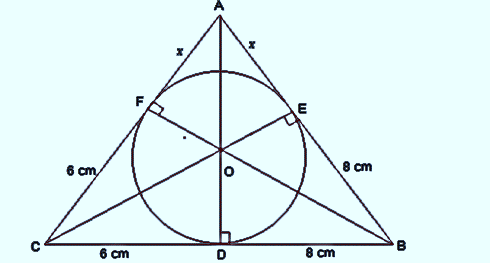In ΔABC,
We know that Length of two tangents drawn from the same point to the circle are equal. Therefore,
CF = CD = 6cm
BE = BD = 8cm
AE = AF = x
Now Side can be calculated as,
AB = AE + EB = x + 8
BC = BD + DC = 8 + 6 = 14
CA = CF + FA = 6 + x
Now semi perimeter of Triangle ABC
s = (AB + BC + CA)/2
= (x + 8 + 14 + 6 + x)/2
= (28 + 2x)/2
= 14 + x
Area of ΔABC = √s (s - a)(s - b)(s - c)
= √(14 + x) (14 + - 14)(14 + - x - 6)(14 + - x - 8)
= √(14 + x) (x)(8)(6)
= √(14 + x) 48 x ... (i)
Now Area of ΔABC can also be calculated as
= ΔOAC + ΔOAB + ΔOBC
= [(1/2×OF×AC) + (1/2×BC×OD) + (1/2×AB×OE)]  [Area of triangle = ½ Height X Base]
Now OD=OE=OF=4cm
=1/2 (4+ 24 + 32) = 56 + 4... (ii)
Equating equation (i) and (ii) we get,
√(14 + x) 48 = 56 + 4x
√(14 + x) 48 = 4(14 + x)
48x =4√(14 + x)
Squaring both sides,
48x = 16 (14 + x)
x = 7 cm
Hence, AB = x + 8 = 7 + 8 = 15 cm
CA = 6 + x = 6 + 7 = 13 cm
Question 13
Prove that opposite sides of a quadrilateral circumscribing a circle subtend supplementary angles at the centre of the circle.
Solution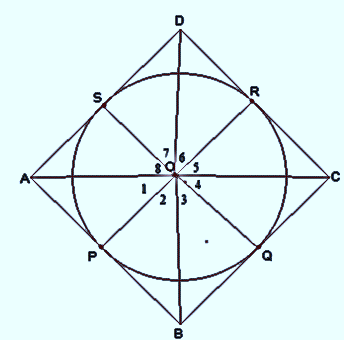Let ABCD be a quadrilateral circumscribing a circle with O such that it touches the circle at point P, Q, R, S. Join the vertices of the quadrilateral ABCD to the center of the circle.
In ΔOAP and ΔOAS,
AP = AS (Tangents from the same point)
OP = OS (Radii of the same circle)
OA = OA (Common side)
By SSS congruence condition
ΔOAP ≅ ΔOAS
∴ ∠POA = ∠AOS
⇒∠1 = ∠8
Similarly we get,
∠2 = ∠3
∠4 = ∠5
∠6 = ∠7
∠1 + ∠2 + ∠3 + ∠4 + ∠5 + ∠6 + ∠7 +∠8 = 360º
(∠1 + ∠8) + (∠2 + ∠3) + (∠4 + ∠5) + (∠6 + ∠7) = 360º
2 ∠1 + 2 ∠2 + 2 ∠5 + 2 ∠6 = 360º
2(∠1 + ∠2) + 2(∠5 + ∠6) = 360º
(∠1 + ∠2) + (∠5 + ∠6) = 180º
∠AOB + ∠COD = 180º
Similarly, we can prove that ∠ BOC + ∠ DOA = 180º
Hence, opposite sides of a quadrilateral circumscribing a circle subtend supplementary angles at the centre of the circle.Go back to Class 10 Main Page using below links

### Practice Question

Question 1 What is $1 - \sqrt {3}$ ?
A) Non terminating repeating
B) Non terminating non repeating
C) Terminating
D) None of the above
Question 2 The volume of the largest right circular cone that can be cut out from a cube of edge 4.2 cm is?
A) 19.4 cm3
B) 12 cm3
C) 78.6 cm3
D) 58.2 cm3
Question 3 The sum of the first three terms of an AP is 33. If the product of the first and the third term exceeds the second term by 29, the AP is ?
A) 2 ,21,11
B) 1,10,19
C) -1 ,8,17
D) 2 ,11,20